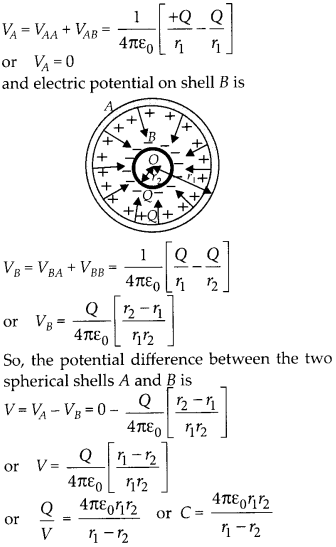Enlightened

# Question 29: NCERT Solutions for 12th Class Physics: Chapter 2-Electrostatic Potential and Capacitance

• 0

Question 29: NCERT Solutions for 12th Class Physics: Chapter 2-Electrostatic Potential and Capacitance

A spherical capacitor consists of two concentric spherical conductors held in position by suitable insulating supports. Show that the capacitance of a spherical capacitor is given byWhere r1 and r2 are the radii of outer and inner spheres, respectively.

Share

1. Solution:

Let + Q be the charge on outer spherical shell A of radius r, and + Q be the charge on inner spherical shell B of radius r2. Then electric potential on shell A isThis gives the capacitance of the spherical capacitor.

Check the complete chapter with solutions.

NCERT Solutions for 12th Class Physics: Chapter 2-Electrostatic Potential and Capacitance

• 0